tracks {ggbio} R Documentation

## Tracks for genomic graphics

### Description

`tracks` is a conventient constructor for bindind graphics as trakcs. You dont' have to worry about adjusting different graphics, `tracks` did that for you. It's NOT just limited to bind genomic tracks, you can use this function to bind any tracks with the same defination of x axis, for example, sets of time series plots you made.

Tracks view is most common way to viewing genome features and annotation data and widely used by most genome browsers. Our assumption is that, most graphics you made with ggbio or by yourself using ggplot2, are almost always sitting on the genomic coordinates or the same x axis. And to compare annotation information along with genome features, we need to align those plots on exactly the same x axis in order to form your hypothesis. This function leaves you the flexibility to construct each tracks separately with worrying your alignments later.

### Usage

```tracks(..., heights, xlim, xlab = NULL, main = NULL,
title = NULL, theme = NULL, fixed = NULL,
track.plot.color = NULL,
track.bg.color = NULL,
main.height = unit(2, "lines"),
scale.height = unit(2, "lines"),
xlab.height = unit(2, "lines"),
label.bg.color =  "white",
label.bg.fill = "gray80",
label.text.color = "black",
label.text.cex = 1,
label.width = unit(2.5, "lines"))
```

### Arguments

 `...` plots of class ggplot, generated from ggplot2 or ggbio. `heights` numeric vector of the same length of passed graphic object to indicate the ratio of each track. `xlim` limits on x. could be `IRanges`, `GRanges`, `numeric` value `xlab` label for x axis. `main` title for the tracks. `title` title for the tracks, alias like main. `theme` theme object used for building tracks, this will set to default, which could be reseted later. `fixed` vector of logical value, indicates whether the scale of passed graphics are fixed or not, useful for attaching unchanged plots to the tracks, like ideogram. Please check utilities section below. `track.plot.color` Vector of characters of length 1 or the same length of passed plots, background color for each track, default is white. `track.bg.color` background color for the whole tracks. `main.height` unit. Height to control the title track height. `scale.height` unit. Height to control the scale track height. `xlab.height` unit. Height to control the xlab track height. `padding` single numeric value or unit, if numeric value, the unit would be "lines" by default. `label.bg.color` track labeling background rectangle border color. `label.bg.fill` track labeling background fill color. `label.text.color` track labeling text color. `label.text.cex` track labeling text size. `label.width` track labeling size.

### Details

`tracks` did following modification for passed plots.

• remove x-axis, ticks, xlab and tile, for each track and add a scale track at bottom. We suppose a new xlab and title would be provided by the `tracks` function for the whole tracks, but we still keep y axis.

• align x-scale limits to make sure every plots sitting on exactly the same x scale.

• squeezing plots together a little bit.

• labeling tracks if names provided, please check utilities section about `labeled` method.

• return a track object. This would allow many features introduced in this manual.

### Value

A `Tracks` object.

### Track class

constructor `tracks` will return a Tracks object, which has following slots.

`grobs`

a `ggplotGrobList` object contains a list of `ggplot` object, which is our passed graphics.

`backup`

a backup of all the slots for holding the original tracks, so users could edit it and reset it back at any time later, and `backup` method will reset the backupped copy.

`ylim`

y limits for each plot.

`labeled`

vector of logical value indicates whether a track is labeled or not, for `labeled` attributes please check utilities section.

`mutable`

vector of logical value indicates whether a track is mutable for theme editing or not, for `mutable` attributes please check utilities section.

`hasAxis`

vector of logical value indicates whether a track has axis or not, for `hasAxis` attributes please check utilities section.

```heights, xlim, xlab, main, title, theme, fixed, track.plot.color, track.bg.color, main.height, scale.height, xlab.height, padding, label.bg.color, label.bg.fill, label.text.color, label.text.cex, label.width```

those slots are described in arguments section for constructor.

### Utilities

`summary(object)`

`fixed(x)`, `fixed(x) <- value`

`x` is the ggplot object, this controls if a track has a fixed x scale or not, if the `fixed` attributes is `TRUE`, then when you pass this plot to a tracks, this plot won't be re-aligned with other tracks and will keep the original x-axis, this allow you to pass some plot like ideogram. `fixed` function will return a logical value

`labeled(x), labeled(x) <- value`

`x` is the ggplot object, if you pass named graphics into `tracks`, it will create the labels on the left for you. Several ways supported to name it. You can pass a list of graphics with names. Or you can use `tracks('name1' = p1, 'name 2' = p2, ...)` with quotes for complicated words or simply `tracks(part1 = p1, part = p2, ...)`.

`mutable(x), mutable(x) <- value`

`x` is the ggplot object, this controls whether a plot in the tracks mutable to theme changing or not, when you use `+` method for Tracks object, add-on edit will only be applied to the the mutable plots.

`bgColor(x), bgColor(x) <- value`

`x` is the ggplot object, this change the background color for single plot shown in the tracks.

`xlim(x), xlim(x) <- value`

when `x` is the numeric value, it calls ggplot2::coord_cartesian(xlim = ...) method, we doesn't use ggplot2::xlim() for the reason it will cut data outside the range, and we believe the best behavior would be zoom-in/out like most browser. when `x` is `IRanges`, `GRanges`, it get the range and passed to ggplot2::coord_cartesian function.

when `x` is Tracks object, `xlim(x)` will return x limits for that tracks. `xlim(x) <- value` replace method only works for Tracks object. `value` could be `numeric`, `IRanges`, `GRanges` object. This will change the x limits associated with tracks.

`+ xlim(obj)`:`obj` is the numeric range, or `IRanges`, `GRanges` object.

`+ coord_cartesian()`: please read manual in ggplot2, this controls both xlim an ylim, only accept numerical range.

`+`

The most nice features about `Tracks` object is the one inherited from ggplot2's components additive features, with `+` method you can use any `theme` object and utilities in ggplot2 package, to add them on a `Tracks` object, for example, if x is our `Tracks` object, `x + theme` would apply theme to any plots in the tracks except those are immutable.

### Backup and reset

reset(obj)

`obj` is the Tracks object, this reset the tracks back to original or backuped version.

backup(obj)

`obj` is the Tracks object, this clear previous backup and use current setting for a new backup.

update(obj, xlim)

`obj` is the Tracks object, this allow a quick tweak with xlim when the plot is shown on your screen.

### Author(s)

Tengfei Yin

`align.plots`

### Examples

``````## make a simulated time series data set
df1 <- data.frame(time = 1:100, score = sin((1:100)/20)*10)
p1 <- qplot(data = df1, x = time, y = score, geom = "line")
df2 <- data.frame(time = 30:120, score = sin((30:120)/20)*10, value = rnorm(120-30 + 1))
p2 <- ggplot(data = df2, aes(x = time, y = score)) +
geom_line() + geom_point(size = 4, aes(color = value))
## check p2
p1
``````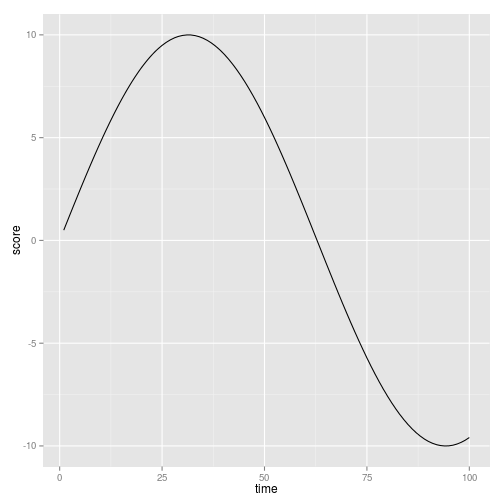``````## check p2
p2
``````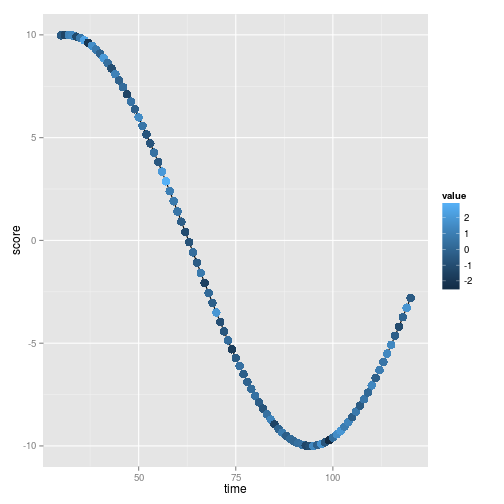``````## binding
tracks(p1, p2)
``````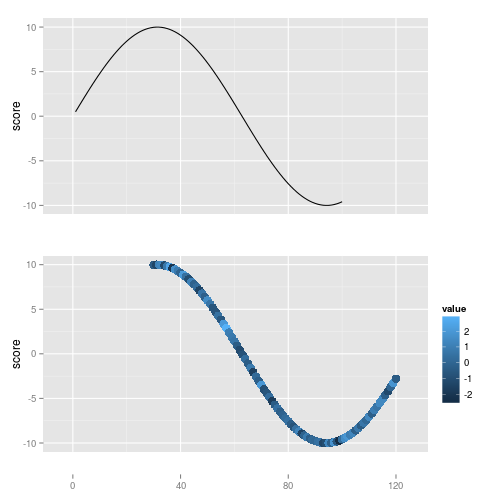``````## or
tks <- tracks(p1, p2)
tks
````````````## labeling: default labeling a named graphic
## simply pass a name with it
tracks(time1 = p1, time2 = p2)
``````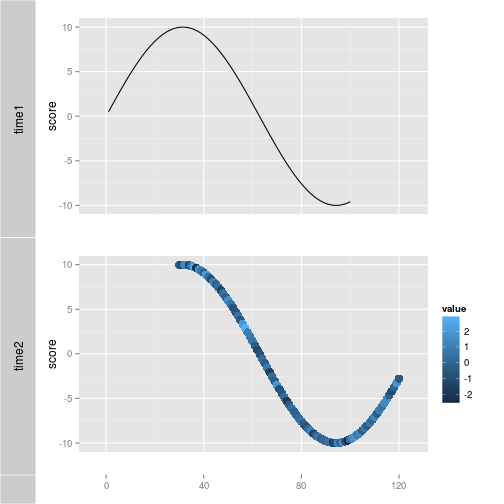``````## or pass a named list with it
lst <- list(time1 = p1, time2 = p2)
tracks(lst)
````````````## more complicated case please use quotes
tracks(time1 = p1, "second time" = p2)
``````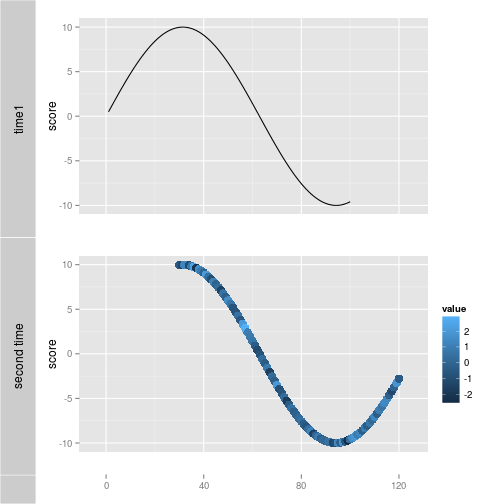``````## set heights
tracks(time1 = p1, time2 = p2, heights = c(1, 3))
``````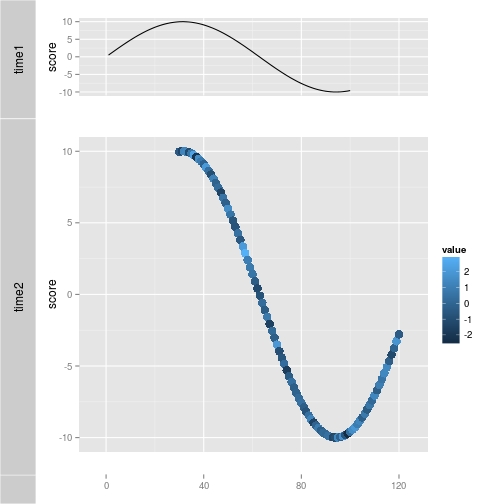``````## if you want to disable label arbitrarily
## default label is always TRUE
labeled(p2)
``````
``````##  TRUE
``````
``````labeled(p2) <- FALSE
## set labeled to FALSE, remove label even the plot has a name
tracks(time1 = p1, time2 = p2)
``````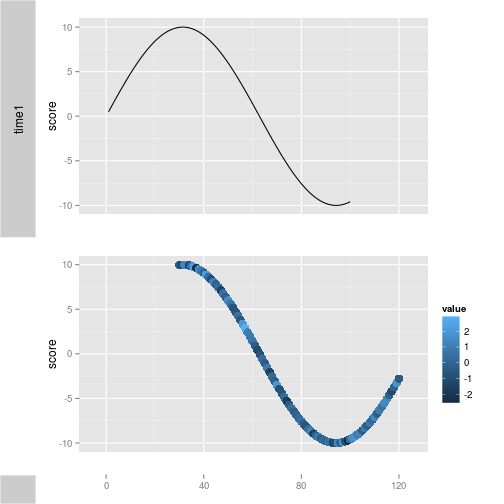``````labeled(p2) <- TRUE
## fix a plot, not synchronize with other plots
p3 <- p1
## default is always FALSE
fixed(p3)
``````
``````##  FALSE
``````
``````## set to TRUE
fixed(p3) <- TRUE
fixed(p3)
``````
``````##  TRUE
``````
``````tracks(time1 = p1, time2 = p2, "time3(fixed)" = p3)
``````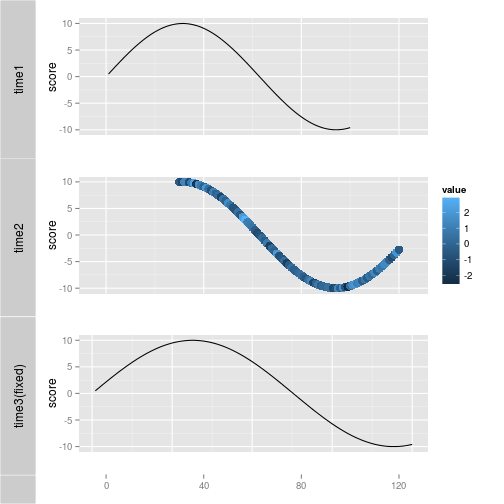``````fixed(p3) <- FALSE
## otherwise you could run
tracks(time1 = p1, time2 = p2, "time3(fixed)" = p3, fixed = c(FALSE, FALSE, TRUE))
````````````## control axis
hasAxis(p1)
``````
``````##  FALSE
``````
``````hasAxis(p1) <- TRUE
tracks(time1 = p1, time2 = p2)
``````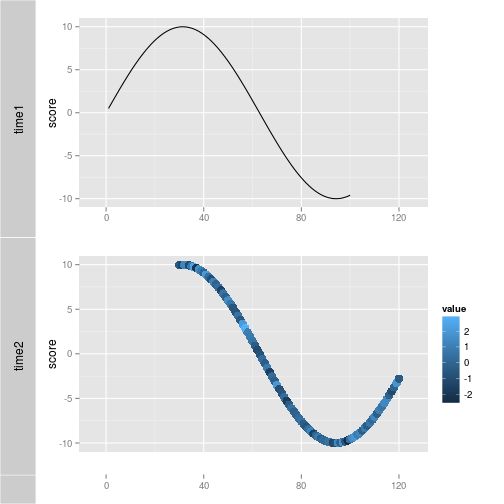``````# set it back
hasAxis(p1) <- FALSE
## mutable
mutable(p1)
``````
``````##  TRUE
``````
``````tracks(time1 = p1, time2 = p2) + theme_bw()
``````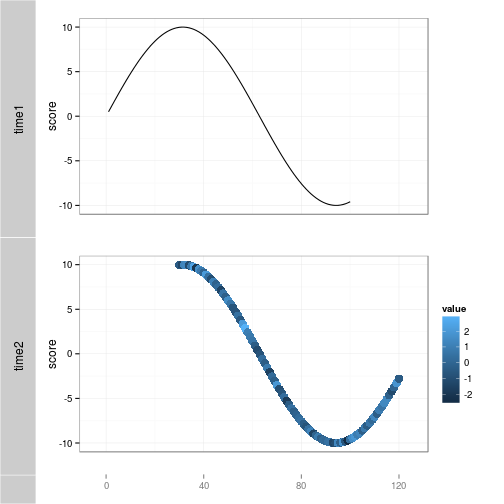``````mutable(p1) <- FALSE
# mutable for "+" method
tracks(time1 = p1, time2 = p2) + theme_bw()
``````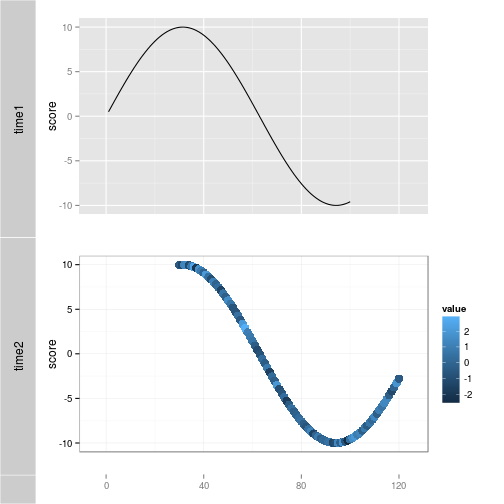``````mutable(p1) <- TRUE
## bgColor
bgColor(p1)
``````
``````##  "white"
``````
``````tracks(time1 = p1, time2 = p2)
````````````bgColor(p1) <- "brown"
# mutable for "+" method
tracks(time1 = p1, time2 = p2)
``````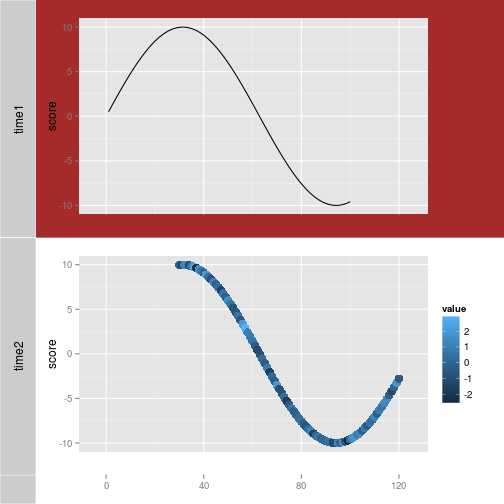``````# set it back
bgColor(p1) <- "white"
## apply a theme to each track
tks <- tracks(time1 = p1, time2 = p2) + theme_bw()
tks
````````````reset(tks)
````````````## store it with tracks
tks <- tracks(time1 = p1, time2 = p2, theme = theme_bw())
tks
````````````tks <- tks + theme_gray()
tks
````````````## reset will be introduced later
reset(tks)
````````````## apply a pre-defiend theme for tracks!
tracks(time1 = p1, time2 = p2) + theme_tracks_sunset()
``````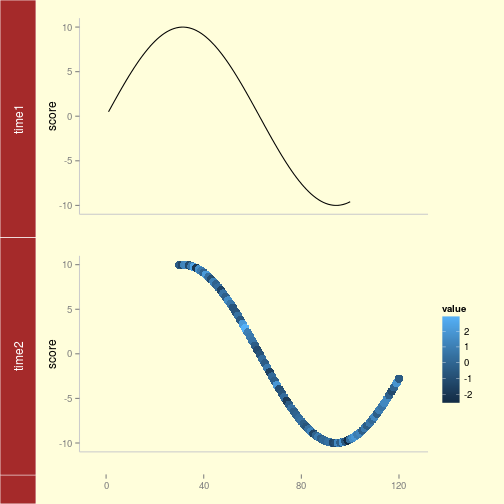`````` tracks(p1, p2) + theme_tracks_sunset()
``````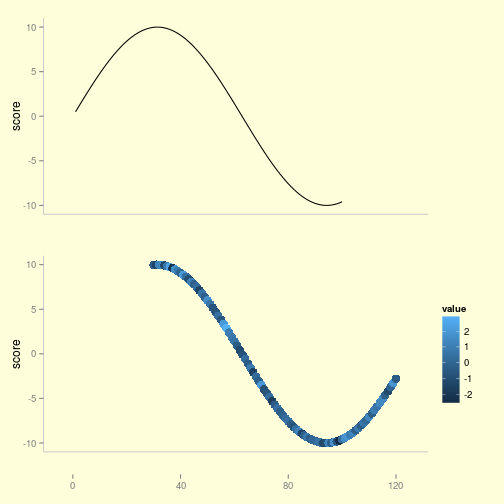``````## change limits
tracks(time1 = p1, time2 = p2) + xlim(c(1, 40))
``````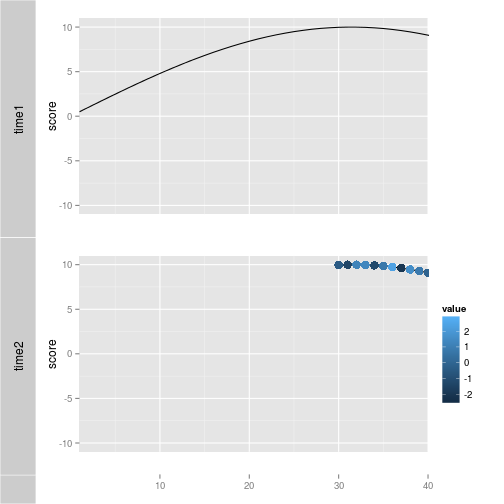``````tracks(time1 = p1, time2 = p2) + xlim(1, 40)
````````````tracks(time1 = p1, time2 = p2) + coord_cartesian(xlim = c(1, 40))
````````````# change y
tracks(time1 = p1, time2 = p2) + xlim(1, 40) + ylim(0, 10)
``````
``````## Warning: Removed 38 rows containing missing values (geom_path).
``````
``````## Warning: Removed 58 rows containing missing values (geom_path).
``````
``````## Warning: Removed 58 rows containing missing values (geom_point).
``````
``````## Warning: Removed 38 rows containing missing values (geom_path).
``````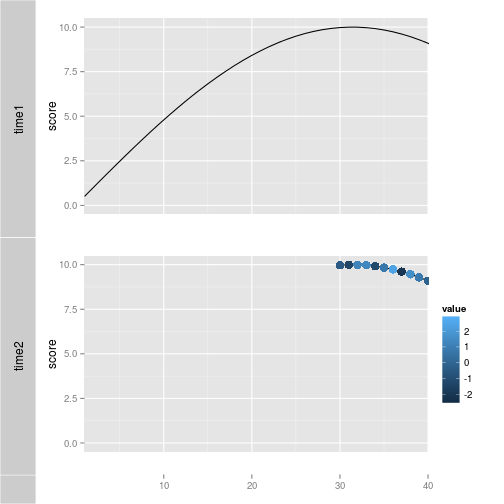``````library(GenomicRanges)
``````
``````## Loading required package: BiocGenerics
``````
``````## Attaching package: 'BiocGenerics'
``````
``````## The following object(s) are masked from 'package:stats':
##
## xtabs
``````
``````## The following object(s) are masked from 'package:base':
##
## anyDuplicated, cbind, colnames, duplicated, eval, Filter, Find, get,
## intersect, lapply, Map, mapply, mget, order, paste, pmax, pmax.int, pmin,
## pmin.int, Position, rbind, Reduce, rep.int, rownames, sapply, setdiff,
## table, tapply, union, unique
``````
``````## Loading required package: IRanges
``````
``````gr <- GRanges("chr", IRanges(1, 40))
# GRanges
tracks(time1 = p1, time2 = p2) + xlim(gr)
````````````# IRanges
tracks(time1 = p1, time2 = p2) + xlim(ranges(gr))
````````````tks <- tracks(time1 = p1, time2 = p2)
xlim(tks)
``````
``````##  -10.9 131.9
``````
``````xlim(tks) <- c(1, 35)
xlim(tks) <- gr
xlim(tks) <- ranges(gr)
## xlab, title
tracks(time1 = p1, time2 = p2, xlab = "time")
``````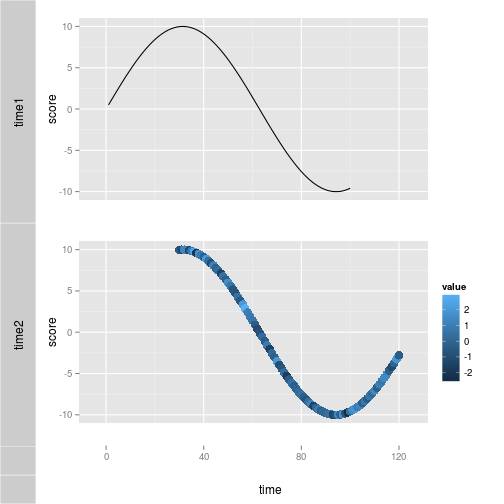``````tracks(time1 = p1, time2 = p2, main = "title")
``````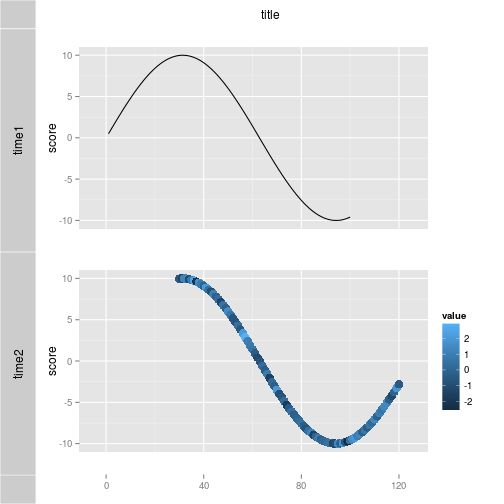``````tracks(time1 = p1, time2 = p2, title = "title")
````````````tracks(time1 = p1, time2 = p2, xlab = "time", title = "title") + theme_tracks_sunset()
``````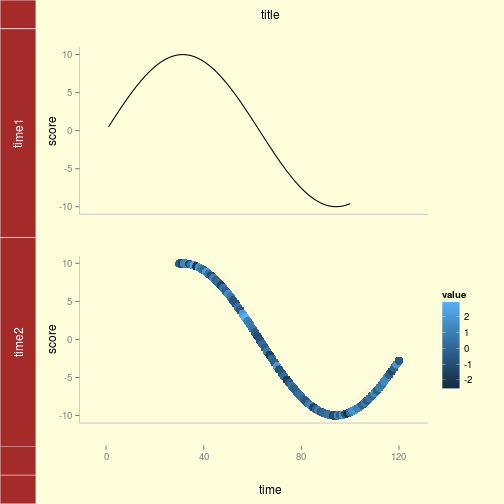``````## backup and restore
tks <- tracks(time1 = p1, time2 = p2)
tks
````````````tks <- tks + xlim(1, 40)
tks
````````````reset(tks)
````````````tks <- tks + xlim(1, 40)
tks
````````````tks <- backup(tks)
tks <- tks + theme_bw()
tks
``````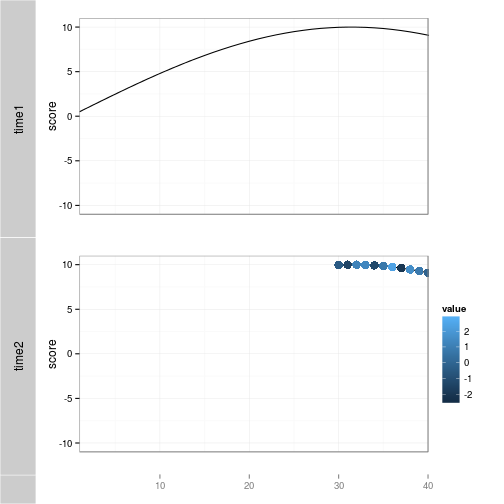``````reset(tks)
````````````## update
## show it on the fly, save your time to re-print
tks <- tracks(time1 = p1, time2 = p2)
tks
````````````update(tks, xlim = c(1, 40))
``````